Mathematics and Computer Science
Volume 1, Issue 3, September 2016, Pages: 44-47

A New Algorithm for Solving Nonlinear Equations by Using Least Square Method

Nasr Al Din Ide

Faculty of Science, Department of Mathematics, Aleppo University, Aleppo, Syria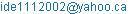Nasr Al Din Ide. A New Algorithm for Solving Nonlinear Equations by Using Least Square Method. Mathematics and Computer Science. Vol. 1, No. 3, 2016, pp. 44-47. doi: 10.11648/j.mcs.20160103.12

Received: August 22, 2016; Accepted: August 31, 2016; Published: September 18, 2016

Abstract: Finding the roots of nonlinear algebraic equations is an important problem in science and engineering, later many methods have been developed for solving nonlinear equations. These methods are given [1-27], in this paper, a new Algorithm for solving nonlinear algebraic equations is obtained by using least square method by fitting a polynomial form of degree two (or parabolic form). This paper compares the present method with the method given by Jutaporn N, Bumrungsak P and Apichat N, 2016 , which was used nonlinear regression method in form of logarithm function. We verified on a number of examples and numerical results obtained show that the present method is faster than the method, which used the logarithm function given by .

Keywords: Nonlinear Algebraic Equations, Least Square Method, Logarithm Function

Contents

1. Introduction

There are several well-known methods for solving nonlinear algebraic equations of the form

f(x)=0 (1)

Where f denote a continuously differentiable on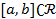and has at least one root α, inSuch as Newton method, Bisection method, Regula Falsi method and several another methods see for example [2-27].

Here we describe a new method by using least square method as a parabola polynomial form of degree two: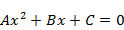(2)

Where A, B and C are the unknown constant.

We used three points (a, f(a)), (b, (f(b)) and (c, f(c)) where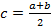, then we find that, this procedure lead us to the root α of equation (1).

2. The Present Method

In beginning, we define three initial points (a, f(a)), (b, (f(b)) and (c, f(c)), now by using least square method we fit the polynomial (2) as suit, let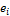is the error or the different value between the true value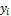and he estimated value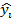, therefore,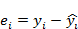(3)

And the sum of square error,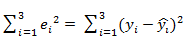(4)

Or,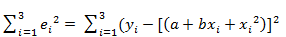(5)

To find A, B, C we will minimize this function, taking the derivative of (5) equal zero with respect to A, B and C we find the three normal equations:

3A+ (a+b+c).B+ (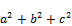).C= f(a)+f(b)+f(c) (6)

(a+b+c).A+ (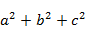).B+ (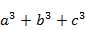).C= a.f(a)+b.f(b)+c.f(c) (7)

().A+().B+(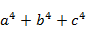).C=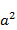.f(a)+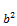.f(b)+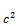.f(c) (8)

Hence, we find A, B and C. Now by solving the equation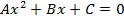we find the two roots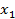andof (2), we chooseorwhich verify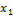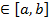or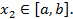3. Algorithm. 1

The present method has 6 steps:

1.  Take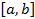is an initial interval, which has at least a root in this interval.

2.  Compute.

3.  Determine the constants A, B and C by solving the system of three linear algebraic equations (6), (7) and (8).

4.  Solve the equation of second degreefor determine the root of (1),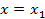or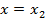which verifyor5.  Replace the intervalwithwhich Contains the root.

6.  Return step (2) until the absolute error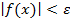.

4. Examples

Example 1. Consider the equation: f(x) =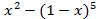= 0. Applying present method, by using Maple program we find the approximate equation of second degree: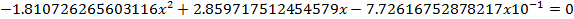In addition, we find for the initial interval [0.1, 1] after 4 iterations, the approximate solution x=0.345954815671666 and the error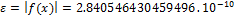.

This result is better than the result given by  which give the root x for 6 iterations with error. Figure 1 illustrate the plots of approximate solution of the equationMoreover, exact solution of the equation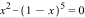for example 1, in the interval [0.1, 1].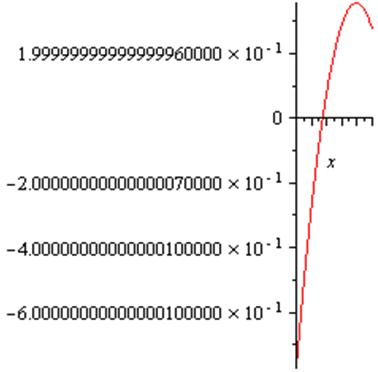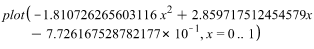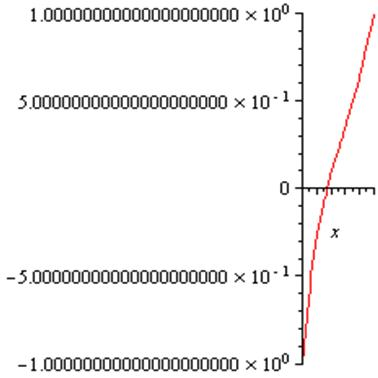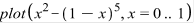Fig. 1. The plot of approximate solution. Exact solution for example 1.

Example 2. Consider the equation: f(x) == 0. Applying present method, by using Maple program we find the approximate equation of second degree: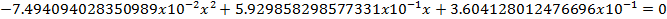In addition, we find for the initial interval [0.1, 1] after four iterations, the approximate solution x=0.5671432901053765 and the error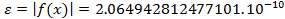.

This result is better than the result given by  which give the root x for seven iterations with error. Figure 2 illustrate the plots of approximate solution of the equationMoreover, exact solution of the equation= 0 for example 1, in the interval [0.1, 1].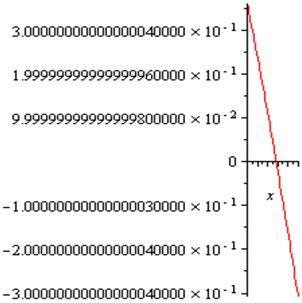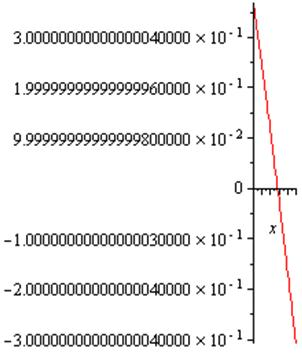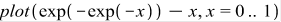Fig. 2. The plot of approximate solution. Exact solution for example 2.

Figure 2 illustrate that, in the interval [0, 1] we have the same graph of the exact solution and the solution given by present method.

Example 3. Consider the equation: f(x) == 0. Applying present method, by using Maple program we find the approximate equation of second degree: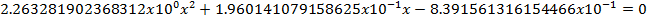In addition, we find for the initial interval [0.1, 1] after four iterations, the approximate solution x=0.5671432904097837 and the error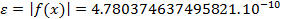.

This result is better than the result given by  which give the root x for six iterations with error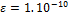. Figure 3 illustrate the plots of approximate solution of the equationMoreover, exact solution of the equation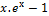= 0 example 1, in the interval [0.1, 1].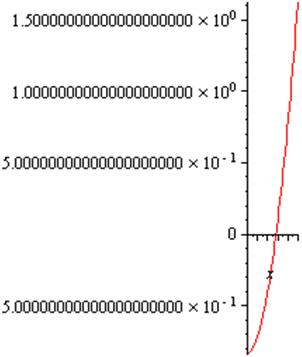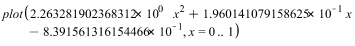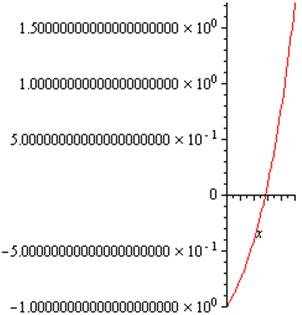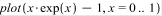Fig. 3. The plot of approximate solution. Exact solution for example 3.

5. Conclusion

The present paper suggests a new algorithm for solving nonlinear algebraic equations; this method presented by equations (6), (7), (8) and by the algorithm 1. Numerical examples show that this method is remarkably effective for solving nonlinear algebraic equations and it is much faster than the method given by , 2016, which was used nonlinear regression method in form of logarithm function.

References

1. Jutaporn N, Bumrungsak P and Apichat N, A new method for finding Root of Nonlinear Equations by using Nonlinear Regression, Asian Journal of Applied Sciences, Vol 03-Issue 06, 2015: 818-822.
2. J. F. Traub,"Iterative Methods for the Solution of Equations", Prentice Hall, Englewood Cliffs, N. J., 1964.
3. Neamvonk A., "A Modified Regula Falsi Method for Solving Root of Nonlinear Equations", Asian Journal of Applied Sciences, vol. 3, no. 4, pp. 776-778, 2015.
4. N. Ide, (2008). A new Hybrid iteration method for solving algebraic equations, Journal of Applied Mathematics and Computation, Elsevier Editorial, Amsterdam, 195, Netherlands, 772-774.
5. N. Ide, (2008). On modified Newton methods for solving a nonlinear algebraic equations, Journal of Applied Mathematics and Computation, Elsevier Editorial, Amsterdam, Netherlands.
6. N. Ide, (2013). Some New Type Iterative Methods for Solving Nonlinear Algebraic Equation", World applied sciences journal, 26 (10); 1330-1334, 2013.
7. M. Javidi, 2007, Iterative Method to Nonlinear Equations, Journal of Applied Mathematics and Computation, Elsevier Editorial, Amsterdam, 193, Netherlands, 360-365.
8. J. H. He (2003). A new iterative method for solving algebraic equations. Appl. Math. Comput. 135: 81-84.
9. M. Javidi, (2009). Fourth-order and fifth-order iterative methods for nonlinear algebraic equations. Math. Comput. Model. 50: 66-71.
10. M. Basto M, V. Semiao, FL. Calheiros (2006). A new iterative method to compute nonlinear equations. Appl. Math. Comput. 173: 468-483.
11. C. Chun (2006). A new iterative method for solving nonlinear equations. Appl. Math. Comput. 178: 415-422.
12. MA. Noor (2007). New family of iterative methods for nonlinear equations. Appl. Math. Compute. 190: 553-558.
13. MA. Noor, KI. Noor, ST. Mohyud-Din, A. Shabbir, (2006). An iterative method with cubic convergence for nonlinear equations. Appl. Math. Comput. 183: 1249-1255.
14. W. Bi, H. Ren, Q. Wu (2009). Three-step iterative methods with eighth-order convergence for solving nonlinear equations. J. Comput. Appl. Math. 225: 105-112.
15. W. Bi, H. Ren, Q. Wu (2009). A new family of eighth-order iterative methods for solving nonlinear equations. Appl. Math. Comput. 214: 236-245.
16. K. Jisheng, L. Yitian, W. Xiuhua (2007). A composite fourth-order iterative method for solving non-linear equations. Appl. Math. Comput. 184: 471-475.
17. N. IDE Some New Iterative Algorithms by Using Homotopy Perturbation Method for Solving Nonlinear Algebraic Equations, 2015, Asian Journal of Mathematics and Computer Research, (AJOMCOR), International Knowledge Press, Vol. 5, Issue 3, 2015.
18. C. Chun, Construction of Newton-like iteration methods for solving nonlinear equations, Numerical Mathematics 104, (2006), 297-315.
19. M. Frontini, and E. Sormani, Some variant of Newton's method with third-order convergence, Applied Mathematics and Computation 140, (2003), 419-426.
20. M. Frontini, and E. Sormani, Third-order methods from quadrature formulae for solving systems of nonlinear equations, Applied Mathematics and Computation 149, (2004), 771-782.
21. J. H. He, Newton-like iteration method for solving algebraic equations, Communications on Nonlinear Science and Numerical Simulation 3, (1998) 106-109.
22. H. H. H. Homeier, A modified Newton method with cubic convergence: the multivariate case, Journal of Computational and Applied Mathematics 169, (2004), 161-169.
23. H. H. H. Homeier, On Newton-type methods with cubic convergence, Journal of Computational and Applied Mathematics 176, (2005), 425-432.
24. J. Kou, Y. Li, and X. Wang, A modification of Newton method with third-order convergence, Applied Mathematics and Computation 181, (2006), 1106-1111.
25. A. Y. Ozban, Some new variants of Newton's method, Applied Mathematics Letters 17, (2004), 677-682.
26. J. F. Traub, Iterative Methods for the Solution of Equations, Chelsea Publishing Company, New York, 1982.
27. S. Weerakoon, and G. I. Fernando, A variant of Newton's method with accelerated third-order convergence, Applied Mathematics Letters 17, (2000), 87-93.

 Contents 1. 2. 3. 4. 5.
Article ToolsAbstractPDF(756K)